JEE  >  Competition Level Test: Application Of Derivative- 1

# Competition Level Test: Application Of Derivative- 1

Test Description

## 30 Questions MCQ Test Mathematics For JEE | Competition Level Test: Application Of Derivative- 1

Competition Level Test: Application Of Derivative- 1 for JEE 2023 is part of Mathematics For JEE preparation. The Competition Level Test: Application Of Derivative- 1 questions and answers have been prepared according to the JEE exam syllabus.The Competition Level Test: Application Of Derivative- 1 MCQs are made for JEE 2023 Exam. Find important definitions, questions, notes, meanings, examples, exercises, MCQs and online tests for Competition Level Test: Application Of Derivative- 1 below.
Solutions of Competition Level Test: Application Of Derivative- 1 questions in English are available as part of our Mathematics For JEE for JEE & Competition Level Test: Application Of Derivative- 1 solutions in Hindi for Mathematics For JEE course. Download more important topics, notes, lectures and mock test series for JEE Exam by signing up for free. Attempt Competition Level Test: Application Of Derivative- 1 | 30 questions in 60 minutes | Mock test for JEE preparation | Free important questions MCQ to study Mathematics For JEE for JEE Exam | Download free PDF with solutions
 1 Crore+ students have signed up on EduRev. Have you?
Competition Level Test: Application Of Derivative- 1 - Question 1

### The two curves x3 - 3xy2 + 2 = 0 and 3x2 y - y3 - 2 = 0

Detailed Solution for Competition Level Test: Application Of Derivative- 1 - Question 1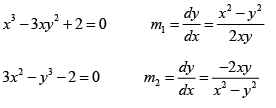m1m2 = -1 ⇒ Curves are orthogonal

Competition Level Test: Application Of Derivative- 1 - Question 2

### The greatest value of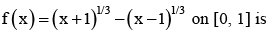Detailed Solution for Competition Level Test: Application Of Derivative- 1 - Question 2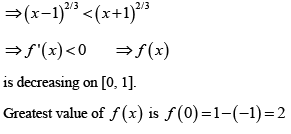Competition Level Test: Application Of Derivative- 1 - Question 3

### The function f (x) = cot-1 x+ x increases in the interval

Detailed Solution for Competition Level Test: Application Of Derivative- 1 - Question 3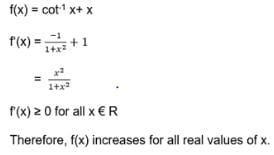Competition Level Test: Application Of Derivative- 1 - Question 4

The real number x (x > 0) when added to its reciprocal gives the minimum sum at x equals

Detailed Solution for Competition Level Test: Application Of Derivative- 1 - Question 4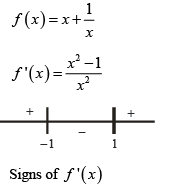Minimum occurs at x = 1

Competition Level Test: Application Of Derivative- 1 - Question 5

If the function f (x) = 2x3 - 9ax2 + 12a2x + 1, where a > 0 , attains its maximum and minimum at p and q respectively such that p² = q, then ‘a’ equals

Detailed Solution for Competition Level Test: Application Of Derivative- 1 - Question 5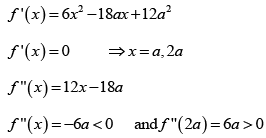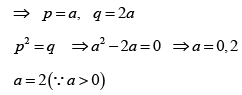Competition Level Test: Application Of Derivative- 1 - Question 6

If 2a + 3b + 6c = 0, then at least one root of the equation ax2 + bx + c = 0 lies in the interval

Detailed Solution for Competition Level Test: Application Of Derivative- 1 - Question 6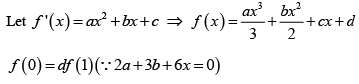By Roll’s theorem, at least one root of f ' (x) =0 lies in (0, 1)

Competition Level Test: Application Of Derivative- 1 - Question 7

A point on the parabola y2 = 18x at which the ordinate increases at twice the rate of the abscissa, is

Detailed Solution for Competition Level Test: Application Of Derivative- 1 - Question 7

y² = 18 x       .....(i)
Differentiating w.r.t t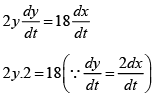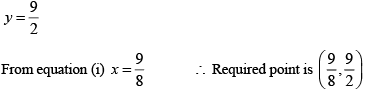Competition Level Test: Application Of Derivative- 1 - Question 8

The normal to the curve  x = a (1 + cos θ) , y = a sin θ at 'θ' always passes through the fixed point

Detailed Solution for Competition Level Test: Application Of Derivative- 1 - Question 8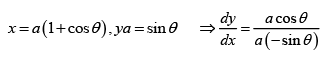Equation of normal at point (a(1 + cos θ) ,a sin θ) is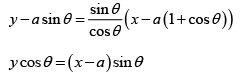It is clear that normal passes through fixed point (a, 0)

Competition Level Test: Application Of Derivative- 1 - Question 9

Angle between the tangents to the curve y = x2 - 5x + 6 at the points (2, 0) and (3, 0) is

Detailed Solution for Competition Level Test: Application Of Derivative- 1 - Question 9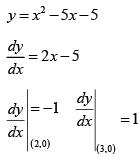Product of slopes = -1 ⇒ angle between tangents is  π/2

Competition Level Test: Application Of Derivative- 1 - Question 10

A function is matched below against an interval where it is supposed to be increasing, Which of the following pairs is incorrectly matched?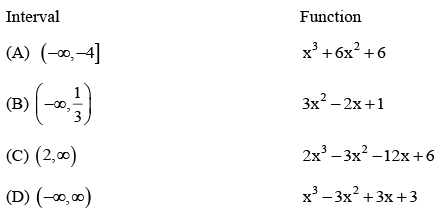Detailed Solution for Competition Level Test: Application Of Derivative- 1 - Question 10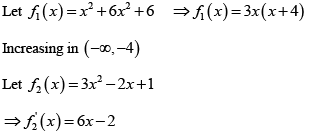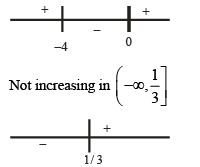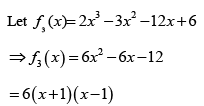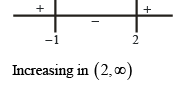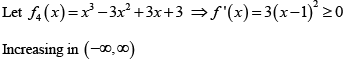Competition Level Test: Application Of Derivative- 1 - Question 11

A spherical iron ball 10 cm in radius is coated with a layer of ice of uniform thickness that melts at a rate of 50 cm³/min. When the thickness of ice is 15 cm, then the rate at which the thickness of ice decreases, is

Detailed Solution for Competition Level Test: Application Of Derivative- 1 - Question 11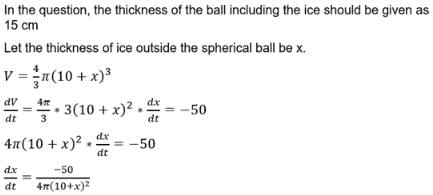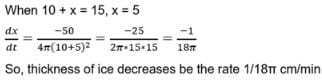Competition Level Test: Application Of Derivative- 1 - Question 12

Area of the greatest rectangle that can be inscribed in the ellipse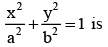Detailed Solution for Competition Level Test: Application Of Derivative- 1 - Question 12

Any point on ellipse is P (x, y) = (a cosθ, b sinθ)
Area of rectangle = 4ab cosθ sinθ
= 2ab sinθ
∴ Maximum area = 2ab.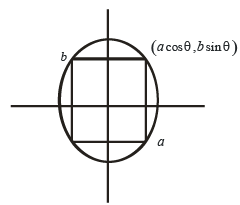Competition Level Test: Application Of Derivative- 1 - Question 13

Let f (x) be differentiable for all x. If f (1) =-2 and f '(x) > 2 for x ∈ (1, 6) then

Detailed Solution for Competition Level Test: Application Of Derivative- 1 - Question 13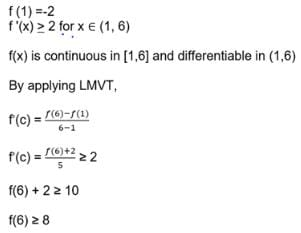Competition Level Test: Application Of Derivative- 1 - Question 14

The normal to the curve x = a (cos θ + θ sin θ), y = a (sin θ - θ cos θ) at any point 'θ' is such that

Detailed Solution for Competition Level Test: Application Of Derivative- 1 - Question 14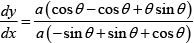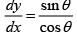Equation of normal is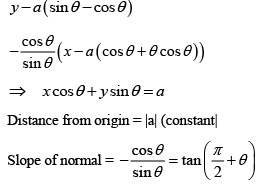⇒ Angle made with x – axis is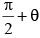Competition Level Test: Application Of Derivative- 1 - Question 15

The function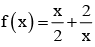has a local minimum at

Detailed Solution for Competition Level Test: Application Of Derivative- 1 - Question 15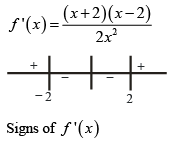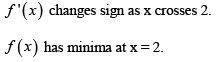Competition Level Test: Application Of Derivative- 1 - Question 16

A value of c for which the conclusion of Mean value Theorem holds for the function f (x) = loge x on the interval [1, 3] is

Detailed Solution for Competition Level Test: Application Of Derivative- 1 - Question 16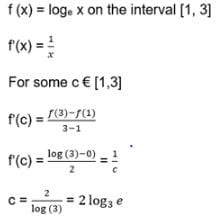Competition Level Test: Application Of Derivative- 1 - Question 17

The function f (x) =  tan-1 (sin x cos x) is an increasing function in

Detailed Solution for Competition Level Test: Application Of Derivative- 1 - Question 17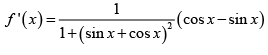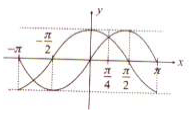Competition Level Test: Application Of Derivative- 1 - Question 18

Suppose the cubic x3 - px + q = 0 has three distinct real roots where p > 0 and q > 0. Then, which one of the following holds?

Detailed Solution for Competition Level Test: Application Of Derivative- 1 - Question 18

Graph of y = x2 - px + q cuts x-axis at three distinct pints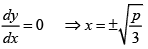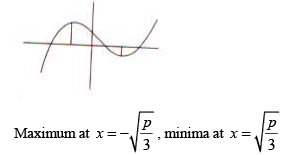Competition Level Test: Application Of Derivative- 1 - Question 19

The shortest distance between the line y - x= 1 and the curve x =y2 is

Detailed Solution for Competition Level Test: Application Of Derivative- 1 - Question 19

Condition for shortest distance is slope of tangent to x = y² must be same as slope of line y = x + 1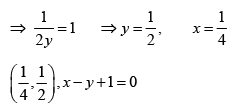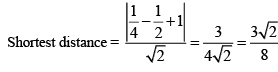Competition Level Test: Application Of Derivative- 1 - Question 20

Given p (x) = x4 + ax3 + bx2 + cx + d such that x = 0 is the only real root of p' (x) = 0 . If p (-1) < p (1) , then in the interval [-1,1]

Detailed Solution for Competition Level Test: Application Of Derivative- 1 - Question 20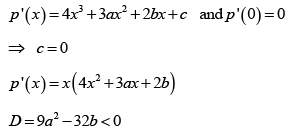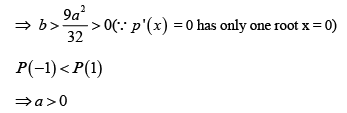p ' (x) has only one change of sign. ⇒ x = 0 is a point of minima.
P(-1) =1- a + b + d P(0) = d
P(1) =1+ a + b + d
⇒ P (-1) < P (1) , P (0) < P (1) , P (-1) > P ( 0)
⇒ P (-1) is not minimum but P(1) is maximum

Competition Level Test: Application Of Derivative- 1 - Question 21

The equation of the tangent to the curve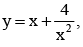that is parallel to the x-axis is

Detailed Solution for Competition Level Test: Application Of Derivative- 1 - Question 21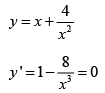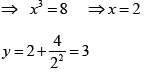(2, 3) is point of contact thus y = 3 is tangent

Competition Level Test: Application Of Derivative- 1 - Question 22

Let f : R → R be defined by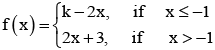If f has a local minimum at x = - 1, then a possible value of k is

Detailed Solution for Competition Level Test: Application Of Derivative- 1 - Question 22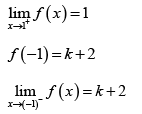∴ f has a local minimum at x = - 1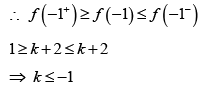Possible value of k is – 1

Competition Level Test: Application Of Derivative- 1 - Question 23

Let f : R → R be a continuous function defined by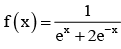Statement-1 :Statement -2 :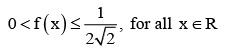Detailed Solution for Competition Level Test: Application Of Derivative- 1 - Question 23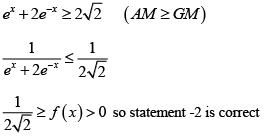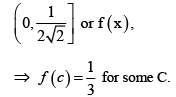Hence correction option is (d)

Competition Level Test: Application Of Derivative- 1 - Question 24

The normal to the curve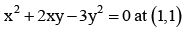Detailed Solution for Competition Level Test: Application Of Derivative- 1 - Question 24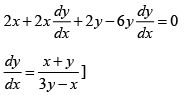Slope of normal at (1, 1) = - 1
∴ Equation of normal y -1 = -1(x -1)
y +x= 2
Solving we get point as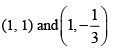Competition Level Test: Application Of Derivative- 1 - Question 25

Consider the function, f (x) =| x - 2 | + | x - 5 | .x ∈ R
Statement -1 : f '(4) = 0
Statement -2 : f is continous in [2, 5], differentiable in (2, 5) and f (2) = f (5)

Detailed Solution for Competition Level Test: Application Of Derivative- 1 - Question 25

f (x) is constant is [2, 5]

Competition Level Test: Application Of Derivative- 1 - Question 26

A spherical balloon is filled with 4500 π cubic meters of helium gas. If a leak in the balloon causes the gas to escape at the rate of 75 π cubic meters per minute, then the rate (in metres per minute) at which the radius of the balloon decreases 49 minutes after the leakage began is

Detailed Solution for Competition Level Test: Application Of Derivative- 1 - Question 26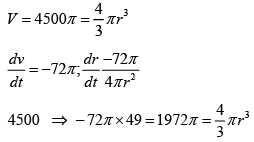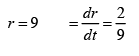Competition Level Test: Application Of Derivative- 1 - Question 27

Let a, b ∈ R be such that the function f given by f (x) = In | x | +bx2 + ax, x ≠ 0 has extreme values at x =-1 and x = 2.

Statement – 1: f has local maximum at x = - 1 and at x = 2.
Statement-2 :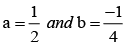Detailed Solution for Competition Level Test: Application Of Derivative- 1 - Question 27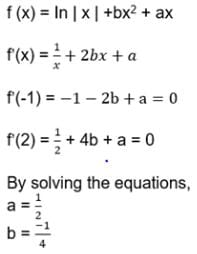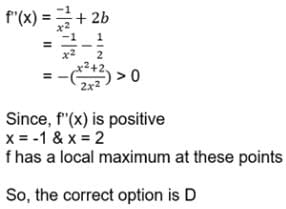Competition Level Test: Application Of Derivative- 1 - Question 28

The real number k for which the equation, 2x3 + 3x + k = 0 has two distinct real roots in [0, 1]

Detailed Solution for Competition Level Test: Application Of Derivative- 1 - Question 28

f ' (x) = 6x2+ 3
⇒ so f(x) is increasing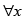equation cannot have 2 distinct roots.

Competition Level Test: Application Of Derivative- 1 - Question 29

The function f (x) = sin4 x+ cos4 x Increasing if

Detailed Solution for Competition Level Test: Application Of Derivative- 1 - Question 29

f '(x) = 4 sin3 x cos x - 4 cos3 x sin x = - sin 4 x for increasing f '(x) >0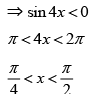Competition Level Test: Application Of Derivative- 1 - Question 30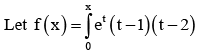dt then f decreasing in the interval

Detailed Solution for Competition Level Test: Application Of Derivative- 1 - Question 30

f ' (x) < 0
ex (x -1) ( x - 2) < 0
1 < x<2

## Mathematics For JEE

130 videos|359 docs|306 tests
Information about Competition Level Test: Application Of Derivative- 1 Page
In this test you can find the Exam questions for Competition Level Test: Application Of Derivative- 1 solved & explained in the simplest way possible. Besides giving Questions and answers for Competition Level Test: Application Of Derivative- 1, EduRev gives you an ample number of Online tests for practice

## Mathematics For JEE

130 videos|359 docs|306 tests(Scan QR code)